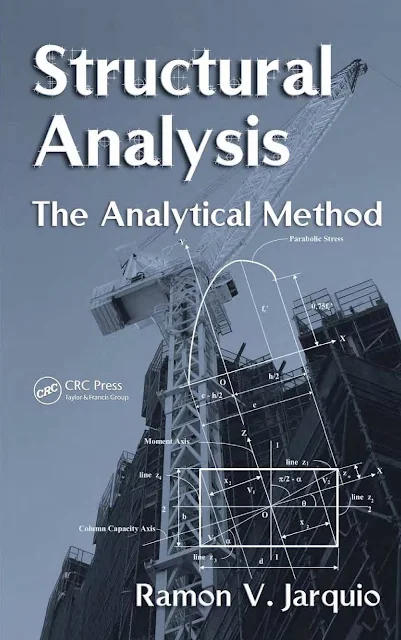# Structural Analysis: The Analytical Method

## Structural Analysis: The Analytical Method

Ramon V. Jarquio, P.E.

Preference :

This book illustrates the analytical procedures for predicting the capacities of circular and rectangular sections in concrete and steel materials. It introduces the capacity axis in the analysis, which is a geometric property not considered in all the current solutions in standard literature. It precludes the use of the current standard interaction formula for biaxial bending, which is a crude and inefficient method. More importantly, the analytical method will prove the necessity of utilizing the capacity axis not only for determining the minimum capacity of a section for biaxial bending but also as a reference axis to satisfy the equilibrium of external and internal forces. Under the current standard interaction formula for biaxial bending, the satisfaction of equilibrium conditions is not possible. Proving the equilibrium condition is the fundamental principle in structural mechanics that every analyst should be able to do.Structural Analysis: The Analytical Method

Content :
• Chapter One: Footing foundation
• Chapter Two: Steel sections
• Chapter Three: Reinforced concrete sections
• Chapter Four: Concrete-filled tube columns

#### Related Posts

Previous
Next Post »